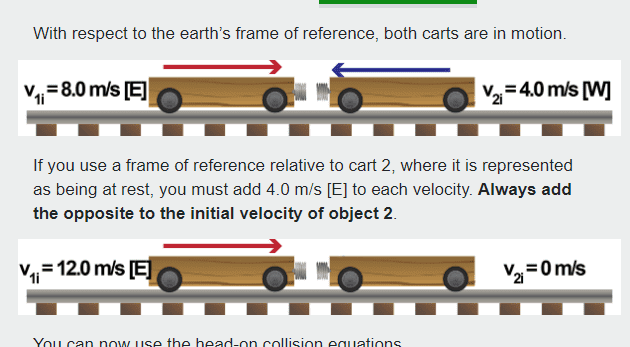• Traced
In summary, the way I learned to solve this was to switch to a frame of reference where one object is stationary.f

#### Traced

Homework Statement
In an elastic head-on collision, a 0.60 kg cart moving at 5.0 m/s [W] collides with a 0.80 kg cart moving at 2.0 m/s [E]. The collision is cushioned by a spring (k = 1200 N/m).
1 Determine the velocity of each cart after the collision.
2 Determine the maximum compression of the spring.
Relevant Equations
n/a
The way I learned to solve this was to switch to a frame of reference where one object is stationary.

given: m1 =0.6kg v1 = 5.0m/s [W], m2 = 0.8kg v2 = 2.0 m/s [E]

Setting v2 to rest by adding 2.0 m/s W to each object

New velocities are v1 = 7.0 m/s [E] and v2 = 0.0m/s

Then using the equations for v1

v1f = V1 (m1 - m2) / (m2 + m1)

= (7.0)(0.6-0.8) / (0.8-0.6)

= -7 m/s

and for v2f

v2f = 2m1(v1) / (m2+m1)

= 2 (0.6) (7.0) / (0.8 + 0.6)
= 6 m/s

Switching back to Earth's frame of reference

v1f = 1.8 m/s
v2f = 4.0 m/s

I get these answers, yet i see other answers online using conservation of energy and they get different answers from me. Am I doing this properly?

•Traced
If you have one object moving at 2.0 m/s and another at 5.0 m/s, you need to add (-2 m/s) to each object to get the 2 m/s object to be at rest. Thus, in that frame the 5 m/s object will have a velocity of 3 m/s.

•Traced
If you have one object moving at 2.0 m/s and another at 5.0 m/s, you need to add (-2 m/s) to each object to get the 2 m/s object to be at rest. Thus, in that frame the 5 m/s object will have a velocity of 3 m/s.

This is what I did in my first attempt but in my lesson it shows an example like this:Which is why in my attempt above I added the 2.0m/s onto the 5.0 m/s. Am I misunderstanding this?

Look at the sentence in bold letters: Always add the opposite to the initial velocity of object 2. In the second example object 2 has velocity -4 m/s so you add the opposite of that which is +4 m/s. In your example object 2 has velocity +2 m/s so you add the opposite of that which is ##\dots##?

•Traced
Look at the sentence in bold letters: Always add the opposite to the initial velocity of object 2. In the second example object 2 has velocity -4 m/s so you add the opposite of that which is +4 m/s. In your example object 2 has velocity +2 m/s so you add the opposite of that which is ##\dots##?
Alright thank you.

This is what I've done now:

Let West be the positive direction

v1f = V1 (m1 - m2) / (m2 + m1)

= (3.0)(0.6-0.8) / (0.8-0.6)
= -0.44 m/s

v2f = 2m1(v1) / (m2+m1)

= 2 (0.6) (3.0) / (0.8 + 0.6)
= 2.57 m/s

So if this is done properly the answers would be v1 = 2.44 m/s [W] and v2 = -4.57 m/s [E] ??

•PeroK
As suggested by @PeroK, if this is done properly then the momentum conservation equation
Pbefore = Pafter should hold. If I put in your numbers,
Pbefore = 0.6*5 + 0.8*2 and Pafter = 0.6*(2.44) + 0.8*(4.57)
Does the equality hold? This is a test you can do yourself.

Alright thank you.

This is what I've done now:

Let West be the positive direction

v1f = V1 (m1 - m2) / (m2 + m1)

= (3.0)(0.6-0.8) / (0.8-0.6)
= -0.44 m/s

v2f = 2m1(v1) / (m2+m1)

= 2 (0.6) (3.0) / (0.8 + 0.6)
= 2.57 m/s

So if this is done properly the answers would be v1 = 2.44 m/s [W] and v2 = -4.57 m/s [E] ??
You need a system here! There's no hope using E and W other than at the beginning and end. The data is:

##m_1 = 0.6 kg##, ##u_1 = 5.0 m/s##, ##m_2 = 0.8 kg##, ##u_2 = - 2.0 m/s##

To get the rest frame of ##m_2## you add ##2 m/s##; to go back to the original frame you add ##-2 m/s##.

PS If W is positive, then it's either ##2 m/s## East or ##-2.0 m/s##, but not ##-2 m/s## East. That's too confusing. One or the other, please!

•Traced
Alternate Method.

Before I present this I would advise PF user Traced that this method involves the use of a formula which one would need (in principle) to derive for oneself before using. The formula is derived using the same well established principles of conservation of momentum and energy which are routinely used for solving collision problems. But - analogous to completing the square in quadratic problems - you can repeatedly complete the square or you can simply use the general formula that derives from completing the square for the general quadratic ##ax^2+bx+c=0## in the first instance.

In a collision the forces acting on the colliding bodies are equal and opposite. Since the "impact time" for both bodies is the same, it follows that they experience equal and opposite impulses. So what we can do is simply calculate that impulse (that's where the formula comes in) and apply it as an equal and opposite change in momentum. Thereby calculate the post-collision momentum for both and divide by their masses to obtain post-collision velocities.

For an elastic collision: $$\Delta P=2\mu \Delta v$$ where ##\mu## is the 'reduced mass' of the colliding bodies: $$\mu=\frac{m_1 m_2}{m_1+m_2}$$ and ##\Delta v## is their relative velocity.

•Traced